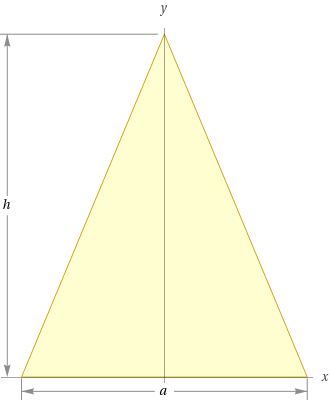Search IntMath
Close

450+ Math Lessons written by Math Professors and Teachers

5 Million+ Students Helped Each Year

1200+ Articles Written by Math Educators and Enthusiasts

Simplifying and Teaching Math for Over 23 Years

# What Are Isosceles Triangles?In geometry, isosceles triangles are three-sided shapes that have two equal sides and two equal angles. The two equal sides are called the legs, and the other side is called the base. Isosceles triangles are some of the most commonly seen shapes in geometry, as they can be found in many everyday objects and in nature.

## Properties of Isosceles Triangles

Isosceles triangles have several important properties that make them stand out from other triangle geometry shapes. First, the two legs of an isosceles triangle are always congruent, meaning they have the same length. This means that the two angles opposite the legs are also congruent, meaning they have the same measure. This makes isosceles triangles unique, as they are the only triangle geometry shape that has two equal sides and two equal angles.

Furthermore, an isosceles triangle has a line of symmetry, meaning that it can be divided into two equal parts. This line of symmetry is the perpendicular line that runs through the midpoint of the base. Isosceles triangles also have a vertex angle, which is the angle between the two equal sides. The vertex angle is always larger than either of the other two angles.

## Uses of Isosceles Triangles

Isosceles triangles are used in a variety of applications, from architecture to engineering. For example, isosceles triangles are often used in roof designs, as their two equal sides provide a stable platform for the roof. Isosceles triangles can also be used to construct bridges, as their two equal sides provide support for the structure. In addition, isosceles triangles are used in engineering, as they are often used to construct machines and other structures.

Isosceles triangles are also used in mathematics, as they can be used to solve a variety of problems. For example, isosceles triangles can be used to calculate the area of a triangle, as well as the lengths of the sides. In addition, isosceles triangles can be used to calculate the angles of the triangle, as well as the perimeter.

## Practice Problems

1. Find the area of an isosceles triangle with a base of 10 cm and legs of 8 cm.

2. Find the length of the base of an isosceles triangle with an area of 18 cm2 and legs of 6 cm.

3. Find the angles of an isosceles triangle with a base of 8 cm and legs of 10 cm.
Answer: The two equal angles are 45�, and the vertex angle is 90�.

4. Find the perimeter of an isosceles triangle with a base of 10 cm and legs of 8 cm.

5. Find the area of an isosceles triangle with a base of 12 cm and an area of 48 cm2.
Answer: The two equal legs are 11 cm.

6. Find the length of the base of an isosceles triangle with an angle of 60� and legs of 8 cm.

## Summary

In geometry, isosceles triangles are three-sided shapes that have two equal sides and two equal angles. Isosceles triangles have several important properties, such as congruent legs and angles, a line of symmetry, and a vertex angle. Isosceles triangles are often used in architecture, engineering, and mathematics, as they can be used to solve a variety of problems. This article provided a few practice problems to help you understand isosceles triangles and their properties.

## FAQ

### What is an isosceles triangle?

An isosceles triangle is a triangle that has two sides of equal length and two angles of equal measure. The two angles are often referred to as the base angles, and the third angle is called the vertex angle.

### What is the sum of the angles of an isosceles triangle?

The sum of the angles of an isosceles triangle is 180�.

## Problem SolverThis tool combines the power of mathematical computation engine that excels at solving mathematical formulas with the power of GPT large language models to parse and generate natural language. This creates math problem solver thats more accurate than ChatGPT, more flexible than a calculator, and faster answers than a human tutor. Learn More.